§2  Basic calculations in analytic geometry

 graphics Calculation formula [ distance between two points ]on flat surfacein space[ The dividing line segment is the dividing point coordinates of the fixed ratio ]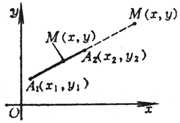( a )( b )   M ( x , y , z ) is the division point of A 1 A 2 , the division ratio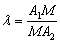on flat surfacein spaceλ > 0 is called inner division and outer division . When λ = 1 , M is the midpoint of A 1 A 2 :, ,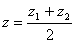graphics Calculation formula [ Area of ​​a triangle on a plane ]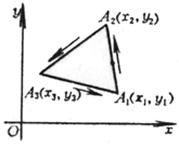A 1 , A 2 , A 3 form a counterclockwise loop S △ =When S △ =0 , the three points of A 1 , A 2 , and A 3 are collinear [ Area of ​​a polygon on a plane ]A 1 , A 2 , … , An form a counterclockwise loop S =When S = 0 , A 1 , A 2 , … , A n n points are collinear [ volume of a tetrahedron in space ]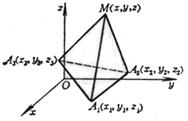right -handed system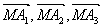V = =When V = 0 , the four points M , A 1 , A 2 , and A 3 are coplanar [ Angle of the dihedral ]M 0 , M 1 , A 1 , A 2 are four non-coplanar points in space, q is the dihedral angle A 1 - M 0 M 1 - A 2 (0 ￡ q ￡ 180 ° )When M 0 is the origin, the positive direction of the x -axis, and the positive direction of the y -axis, thenWhen M 0 is the origin and the positive direction of the x -axis, thengraphics Calculation formula [ Barycentric coordinates of mass point system ]The mass of each particle in space is m i , and the coordinates are ( x i , y i , z i ) i =1, 2, … , n The coordinates of the center of gravity G are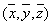where ∑ represents the sum of i from 1 to n